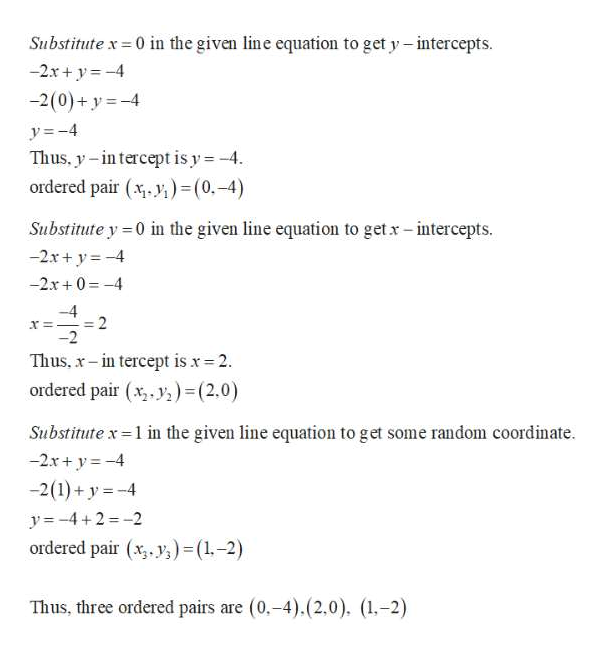# How do I find the x and y intercepts of the line represented by the equation-2x+y= -4?

Question
6 views

How do I find the x and y intercepts of the line represented by the equation-2x+y= -4?

check_circle

Step 1

Note that given equation...help_outlineImage TranscriptioncloseSubstitute x = 0 in the given line equation to get y- intercepts. -2.x+ y = -4 -2(0)+ y =-4 y=-4 Thus, y- in tercept is y=-4. ordered pair (x. y,)=(0.-4) Substitute y = 0 in the given line equation to getx- intercepts. -2x+ y = -4 -2x+0 =-4 -4 = 2 -2 Thus, x- in tercept is x= 2. ordered pair (x,. y;)= (2,0) Substitute x=1 in the given line equation to get some random coordinate. -2x+ y = -4 -2(1) + y = -4 y=-4+2 = -2 ordered pair (x,. y;) = (1.-2) Thus, three ordered pairs are (0,-4).(2.0). (1,-2) fullscreen

### Want to see the full answer?

See Solution

#### Want to see this answer and more?

Solutions are written by subject experts who are available 24/7. Questions are typically answered within 1 hour.*

See Solution
*Response times may vary by subject and question.
Tagged in

### Math